Anzeige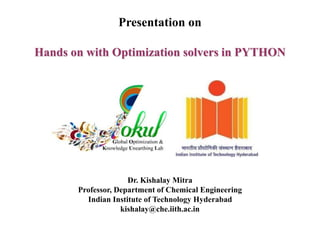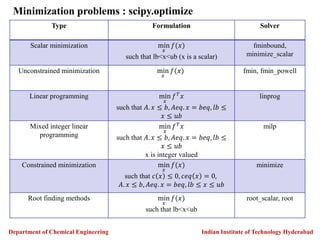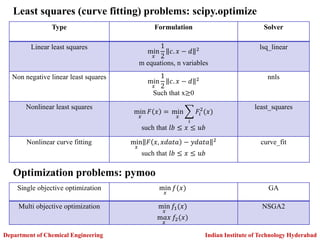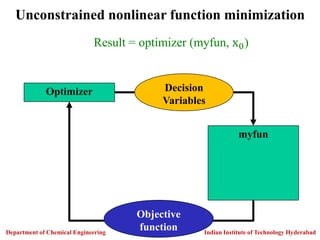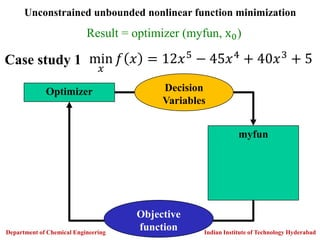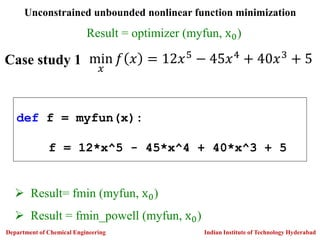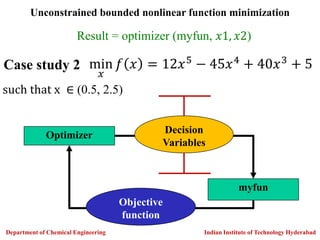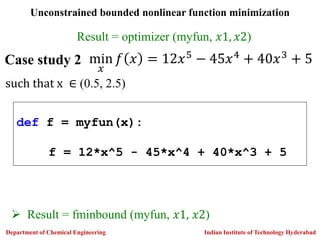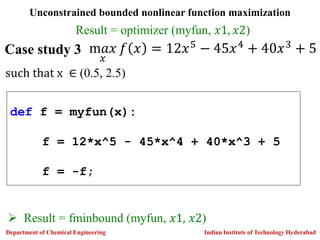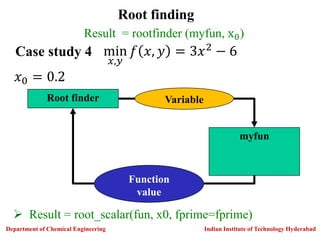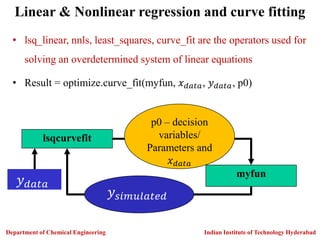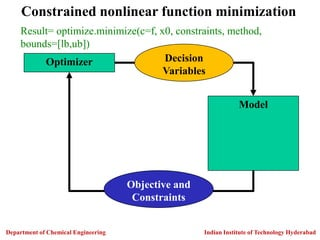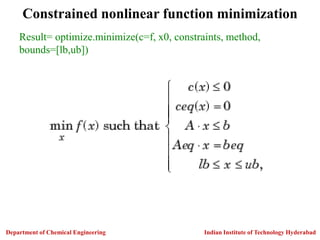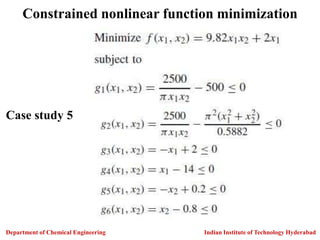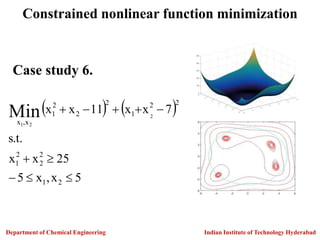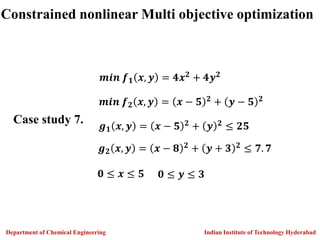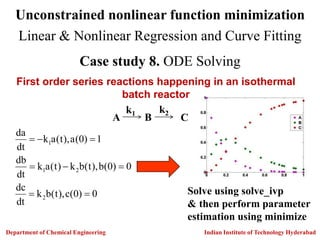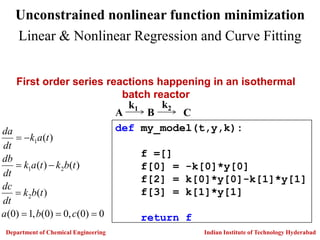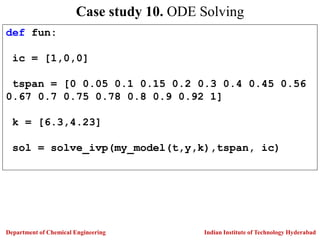1 von 19
Anzeige

### Hands on Optimization in Python (1).pptx

1. Hands on with Optimization solvers in PYTHON Presentation on Dr. Kishalay Mitra Professor, Department of Chemical Engineering Indian Institute of Technology Hyderabad kishalay@che.iith.ac.in
2. Indian Institute of Technology Hyderabad Department of Chemical Engineering Type Formulation Solver Scalar minimization min 𝑥 𝑓(𝑥) such that lb<x<ub (x is a scalar) fminbound, minimize_scalar Unconstrained minimization min 𝑥 𝑓(𝑥) fmin, fmin_powell Linear programming min 𝑥 𝑓𝑇 𝑥 such that 𝐴. 𝑥 ≤ 𝑏, 𝐴𝑒𝑞. 𝑥 = 𝑏𝑒𝑞, 𝑙𝑏 ≤ 𝑥 ≤ 𝑢𝑏 linprog Mixed integer linear programming min 𝑥 𝑓𝑇 𝑥 such that 𝐴. 𝑥 ≤ 𝑏, 𝐴𝑒𝑞. 𝑥 = 𝑏𝑒𝑞, 𝑙𝑏 ≤ 𝑥 ≤ 𝑢𝑏 x is integer valued milp Constrained minimization min 𝑥 𝑓(𝑥) such that 𝑐 𝑥 ≤ 0, 𝑐𝑒𝑞 𝑥 = 0, 𝐴. 𝑥 ≤ 𝑏, 𝐴𝑒𝑞. 𝑥 = 𝑏𝑒𝑞, 𝑙𝑏 ≤ 𝑥 ≤ 𝑢𝑏 minimize Root finding methods min 𝑥 𝑓(𝑥) such that lb<x<ub root_scalar, root Minimization problems : scipy.optimize
3. Department of Chemical Engineering Indian Institute of Technology Hyderabad Type Formulation Solver Linear least squares min 𝑥 1 2 𝑐. 𝑥 − 𝑑 2 m equations, n variables lsq_linear Non negative linear least squares min 𝑥 1 2 𝑐. 𝑥 − 𝑑 2 Such that x≥0 nnls Nonlinear least squares min 𝑥 𝐹 𝑥 = min 𝑥 𝑖 𝐹𝑖 2 (𝑥) such that 𝑙𝑏 ≤ 𝑥 ≤ 𝑢𝑏 least_squares Nonlinear curve fitting min 𝑥 𝐹 𝑥, 𝑥𝑑𝑎𝑡𝑎 − 𝑦𝑑𝑎𝑡𝑎 2 such that 𝑙𝑏 ≤ 𝑥 ≤ 𝑢𝑏 curve_fit Least squares (curve fitting) problems: scipy.optimize Single objective optimization min 𝑥 𝑓(𝑥) GA Multi objective optimization min 𝑥 𝑓1(𝑥) m𝑎𝑥 𝑥 𝑓2(𝑥) NSGA2 Optimization problems: pymoo
4. Optimizer myfun Decision Variables Objective function Unconstrained nonlinear function minimization Result = optimizer (myfun, x0) Indian Institute of Technology Hyderabad Department of Chemical Engineering
5. Case study 1 min 𝑥 𝑓 𝑥 = 12𝑥5 − 45𝑥4 + 40𝑥3 + 5 Optimizer myfun Decision Variables Objective function Result = optimizer (myfun, x0) Unconstrained unbounded nonlinear function minimization Indian Institute of Technology Hyderabad Department of Chemical Engineering
6. def f = myfun(x): f = 12*x^5 - 45*x^4 + 40*x^3 + 5 Case study 1 min 𝑥 𝑓 𝑥 = 12𝑥5 − 45𝑥4 + 40𝑥3 + 5  Result= fmin (myfun, x0)  Result = fmin_powell (myfun, x0) Indian Institute of Technology Hyderabad Department of Chemical Engineering Result = optimizer (myfun, x0) Unconstrained unbounded nonlinear function minimization
7. Case study 2 min 𝑥 𝑓 𝑥 = 12𝑥5 − 45𝑥4 + 40𝑥3 + 5 Optimizer myfun Decision Variables Objective function Result = optimizer (myfun, 𝑥1, 𝑥2) such that x ∈ (0.5, 2.5) Indian Institute of Technology Hyderabad Department of Chemical Engineering Unconstrained bounded nonlinear function minimization
8. def f = myfun(x): f = 12*x^5 - 45*x^4 + 40*x^3 + 5 Case study 2 min 𝑥 𝑓 𝑥 = 12𝑥5 − 45𝑥4 + 40𝑥3 + 5 Result = optimizer (myfun, 𝑥1, 𝑥2) such that x ∈ (0.5, 2.5)  Result = fminbound (myfun, 𝑥1, 𝑥2) Indian Institute of Technology Hyderabad Department of Chemical Engineering Unconstrained bounded nonlinear function minimization
9. def f = myfun(x): f = 12*x^5 - 45*x^4 + 40*x^3 + 5 f = -f; Case study 3 m𝑎𝑥 𝑥 𝑓 𝑥 = 12𝑥5 − 45𝑥4 + 40𝑥3 + 5 such that x ∈ (0.5, 2.5) Indian Institute of Technology Hyderabad Department of Chemical Engineering Unconstrained bounded nonlinear function maximization Result = optimizer (myfun, 𝑥1, 𝑥2)  Result = fminbound (myfun, 𝑥1, 𝑥2)
10. Case study 4 Root finder myfun Variable Function value Result = rootfinder (myfun, x0) Root finding min 𝑥,𝑦 𝑓 𝑥, 𝑦 = 3𝑥2 − 6 𝑥0 = 0.2 Indian Institute of Technology Hyderabad Department of Chemical Engineering  Result = root_scalar(fun, x0, fprime=fprime)
11. • lsq_linear, nnls, least_squares, curve_fit are the operators used for solving an overdetermined system of linear equations • Result = optimize.curve_fit(myfun, 𝑥𝑑𝑎𝑡𝑎, 𝑦𝑑𝑎𝑡𝑎, p0) lsqcurvefit myfun p0 – decision variables/ Parameters and 𝑥𝑑𝑎𝑡𝑎 𝑦𝑠𝑖𝑚𝑢𝑙𝑎𝑡𝑒𝑑 𝑦𝑑𝑎𝑡𝑎 Indian Institute of Technology Hyderabad Department of Chemical Engineering Linear & Nonlinear regression and curve fitting
12. Result= optimize.minimize(c=f, x0, constraints, method, bounds=[lb,ub]) Optimizer Model Decision Variables Objective and Constraints Constrained nonlinear function minimization Indian Institute of Technology Hyderabad Department of Chemical Engineering
13. Result= optimize.minimize(c=f, x0, constraints, method, bounds=[lb,ub]) Constrained nonlinear function minimization Indian Institute of Technology Hyderabad Department of Chemical Engineering
14. Case study 5 Indian Institute of Technology Hyderabad Department of Chemical Engineering Constrained nonlinear function minimization
15.     5 x , x 5 25 x x . t . s 7 x x 11 x x 2 1 2 2 2 1 2 2 1 2 2 2 1 x , x 2 2 1 Min           Constrained nonlinear function minimization Case study 6. Indian Institute of Technology Hyderabad Department of Chemical Engineering
16. Indian Institute of Technology Hyderabad Department of Chemical Engineering 𝒎𝒊𝒏 𝒇𝟏 𝒙, 𝒚 = 𝟒𝒙𝟐 + 𝟒𝒚𝟐 𝒎𝒊𝒏 𝒇𝟐 𝒙, 𝒚 = 𝒙 − 𝟓 𝟐 + 𝒚 − 𝟓 𝟐 𝒈𝟏 𝒙, 𝒚 = 𝒙 − 𝟓 𝟐 + 𝒚 𝟐 ≤ 𝟐𝟓 𝒈𝟐 𝒙, 𝒚 = 𝒙 − 𝟖 𝟐 + 𝒚 + 𝟑 𝟐 ≤ 𝟕. 𝟕 𝟎 ≤ 𝒙 ≤ 𝟓 𝟎 ≤ 𝒚 ≤ 𝟑 Constrained nonlinear Multi objective optimization Case study 7.
17. Unconstrained nonlinear function minimization Linear & Nonlinear Regression and Curve Fitting Case study 8. ODE Solving First order series reactions happening in an isothermal batch reactor 0 ) 0 ( c ), t ( b k dt dc 0 ) 0 ( b ), t ( b k ) t ( a k dt db 1 ) 0 ( a ), t ( a k dt da 2 2 1 1         A B C k1 k2 Solve using solve_ivp & then perform parameter estimation using minimize Indian Institute of Technology Hyderabad Department of Chemical Engineering
18. Unconstrained nonlinear function minimization Linear & Nonlinear Regression and Curve Fitting First order series reactions happening in an isothermal batch reactor 0 ) 0 ( , 0 ) 0 ( , 1 ) 0 ( ) ( ) ( ) ( ) ( 2 2 1 1         c b a t b k dt dc t b k t a k dt db t a k dt da A B C k1 k2 def my_model(t,y,k): f =[] f = -k*y f = k*y-k*y f = k*y return f Indian Institute of Technology Hyderabad Department of Chemical Engineering
19. def fun: ic = [1,0,0] tspan = [0 0.05 0.1 0.15 0.2 0.3 0.4 0.45 0.56 0.67 0.7 0.75 0.78 0.8 0.9 0.92 1] k = [6.3,4.23] sol = solve_ivp(my_model(t,y,k),tspan, ic) Case study 10. ODE Solving Indian Institute of Technology Hyderabad Department of Chemical Engineering
Anzeige